ICSE Class 9 Triangles Solution New Pattern

## ICSE Class 9 Triangles Solution New Pattern By Clarify Knowledge

ICSE Class 9 Logarithms Solution New Pattern 2022

## Chapter 9 - Triangles [Congruency in Triangles] Exercise Ex. 9(A)

Question 1

Which of the following pairs of triangles are congruent? In each case, state the condition of congruency:

(a) In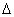ABC andDEF, AB = DE, BC = EF and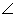B =E.

(b) InABC andDEF,B =E = 90o; AC = DF and BC = EF.

(c) InABC andQRP, AB = QR,B =R andC = P.

(d) InABC andPQR, AB = PQ, AC = PR and BC = QR.

(e) InADC andPQR, BC = QR,A = 90oC =R = 40o andQ = 50o.Solution 1

Question 2

The given figure shows a circle with centre O. P is mid-point of chord AB.

Show that OP is perpendicular to AB.Solution 2

Question 3

The following figure shows a circle with centre O.

If OP is perpendicular to AB, prove that AP = BP.Solution 3

Question 4

In a triangle ABC, D is mid-point of BC; AD is produced upto E so that DE = AD. Prove that:

(i)ABD andECD are congruent.

(ii) AB = CE.

(iii) AB is parallel to EC.Solution 4

Question 5

A triangle ABC hasB =C.

Prove that:

(i) The perpendiculars from the mid-point of BC to AB and AC are equal.

(ii) The perpendiculars form B and C to the opposite sides are equal.Solution 5

Question 6

The perpendicular bisectors of the sides of a triangle ABC meet at I.

Prove that: IA = IB = IC.Solution 6

Question 7

A line segment AB is bisected at point P and through point P another line segment PQ, which is perpendicular to AB, is drawn. Show that: QA = QB.Solution 7

Question 8

If AP bisects angle BAC and M is any point on AP, prove that the perpendiculars drawn from M to AB and AC are equal.Solution 8

Question 9

From the given diagram, in which ABCD is a parallelogram, ABL is al line segment and E is mid point of BC.

Prove that:

(i)DCE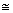LBE

(ii) AB = BL.

(iii) AL = 2DC

Solution 9

Question 10

In the given figure, AB = DB and Ac = DC.

IfABD = 58o,DBC = (2x - 4)o,ACB = y + 15o andDCB = 63o ; find the values of x and y.Solution 10

Question 11

In the given figure: AB//FD, AC//GE and BD = CE; prove that:

(i) BG = DF

(ii) CF = EG

Solution 11

Question 12

In ∆ABC, AB = AC. Show that the altitude AD is median also.Solution 12

Question 13

In the following figure, BL = CM.

Prove that AD is a median of triangle ABC.Solution 13

Question 14

In the following figure, AB = AC and AD is perpendicular to BC. BE bisects angle B and EF is perpendicular to AB.

Prove that :

(i) BD = CD

(ii) ED = EF

Solution 14

Question 15

Use the information in the given figure to prove :

(i) AB = FE

(ii) BD = CF

Solution 15

## Chapter 9 - Triangles [Congruency in Triangles] Exercise Ex. 9(B)

Question 1

On the sides AB and AC of triangle ABC, equilateral triangle ABD and ACE are drawn.

Prove that: (i)CAD =BAE    (ii) CD = BE.Solution 1

Question 2

In the following diagrams, ABCD is a square and APB is an equilateral triangle.

In each case,

(i) Prove that:APDBPC

(ii) Find the angles ofDPC.

Solution 2

Question 3

In the figure, given below, triangle ABC is right-angled at B. ABPQ and ACRS are squares. Prove that:

(i)ACQ andASB are congruent.

(ii) CQ = BS.

Solution 3

Question 4

In aABC, BD is the median to the side AC, BD is produced to E such that BD = DE. Prove that: AE is parallel to BC.Solution 4

Question 5

In the adjoining figure, OX and RX are the bisectors of the angles Q and R respectively of the triangle PQR.

If XS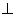QR and XTPQ ; prove that:

(i)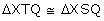(ii) PX bisects angle P.Solution 5

Question 6

In the parallelogram ABCD, the angles A and C are obtuse. Points X and Y are taken on the diagonal BD such that the angles XAD and YCB are right angles.

Prove that: XA = YC.Solution 6

Question 7

ABCD is a parallelogram. The sides AB and AD are produced to E and F respectively, such produced to E and F respectively, such that AB = BE and AD = DF.

Prove that:BECDCF.Solution 7

Question 8

In the following figures, the sides AB and BC and the median AD of triangle ABC are equal to the sides PQ and QR and median PS of the triangle PQR. Prove thatABC andPQR are congruent.

Solution 8

Question 9

In the following diagram, AP and BQ are equal and parallel to each other.

Prove that:

(i)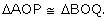(ii) AB and PQ bisect each other.Solution 9

Question 10

In the following figure, OA = OC and AB = BC.

Prove that:

(i)AOB = 90o

(ii)AODCOD

Question 11

The following figure shown a triangle ABC in which AB = AC. M is a point on AB and N is a point on AC such that BM = CN.

Prove that:

(i) AM = AN      (ii)AMCANB

(iii) BN = CM    (iv)BMCCNB

Solution 11

Question 12

In a triangle ABC, AB = BC, AD is perpendicular to side BC and CE is perpendicular to side AB. Prove that : AD = CE.Solution 12

Question 13

PQRS is a parallelogram. L and M are points on PQ and SR respectively such that PL= MR. Show that LM and QS bisect each other.Solution 13

Question 14

In the following figure, ABC is an equilateral triangle in which QP is parallel to AC. Side AC is produced upto point R so that CR = BP.

Prove that QR bisects PC.

Hint: (Show that ∆ QBP is equilateral

⇒ BP = PQ, but BP = CR

⇒ PQ = CR ⇒ ∆ QPM ≅ ∆ RCM) Solution 14

Question 15

In the following figure, ∠A = ∠C and AB = BC. Prove that ΔABD ≅ ΔCBE.

Solution 15

In triangles AOE and COD,

∠A = ∠C  (given)

∠AOE = ∠COD  (vertically opposite angles)

∴ ∠A + ∠AOE = ∠C + ∠COD

⇒180° - ∠AEO = 180° - ∠CDO

⇒ ∠AEO = ∠ CDO  ….(i)

Now, ∠AEO + ∠OEB = 180° (linear pair)

And, ∠CDO + ∠ODB = 180° (linear pair)

∴ ∠AEO + ∠OEB = ∠CDO + ∠ODB

⇒ ∠OEB = ∠ODB [Using (i)]

Now, in ΔABD and ΔCBE,

∠A = ∠C  (given)

AB = BC  (given)

⇒ ΔABD ≅ ΔCBE  (by AAS congruence criterion) Question 16

AD and BC are equal perpendiculars to a line segment AB. If AD and BC are on different sides of AB prove that CD bisects AB.Solution 16

In ΔAOD and ΔBOC,

∠ AOD = ∠ BOC (vertically opposite angles)

∠ DAO = ∠ CBO (each 90°)

∴ ΔAOD ≅ ΔBOC (by AAS congruence criterion)

⇒ AO = BO [cpct]

⇒ O is the mid-point of AB.

Hence, CD bisects AB.Question 17

In ΔABC, AB = AC and the bisectors of angles B and C intersect at point O. Prove that :

(i) BO = CO

(ii) AO bisects angle BAC.Solution 17

In ΔABC,

AB = AC

⇒ ∠B = ∠C (angles opposite to equal sides are equal)

Question 18

In the following figure, AB = EF, BC = DE and ∠B = ∠E = 90°.

Prove that AD = FC.Solution 18

Question 19

A point O is taken inside a rhombus ABCD such that its distance from the vertices B and D are equal. Show that AOC is a straight line.Solution 19

Question 20

In quadrilateral ABCD, AD = BC and BD = CA. Prove that:

(ii) ∠DAB = ∠CBASolution 20

In ΔABD and ΔBAC,